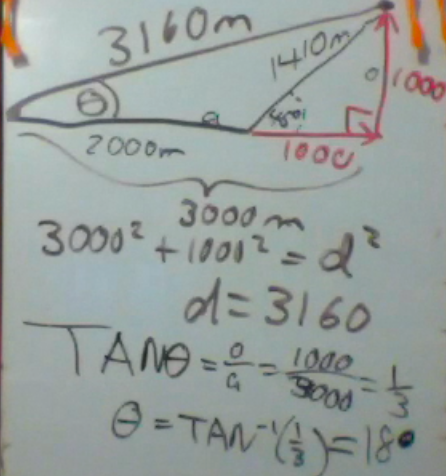## Inverse Trig Function

When trying to find the angle in a right triangle, we can use the inverse trigonometric functions, or arc-trig functions.

Do not confuse this with the reciprocal functions.

An inverse sine function, (also called arcsine)  runs the sine function in reverse.

If SIN θ = x,    then ArcSIN x = θHere is an example where we are given two sides of a triangle.  The hypotenuse is 100, and the opposite side is 86.6  We want to find an unknown angle θ.

Since SIN θ = o/h = 86.6/100 = 0.866 then we take the ArcSIN of 0.866 to get 60°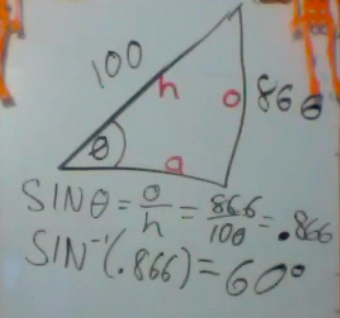Here is a harder vector problem that you will see in the homework.  Let us say you travel for 2000 meters east.  Then you walk 1410 meters Northeast at 45°. You want to find the total displacement.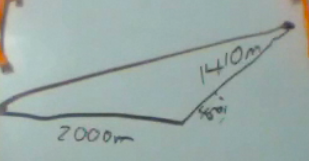First you must break the 1410 into horizontal and vertical components.  Since it is a 45° triangle, we don’t even need trigonometry.  The components are each 1000 m.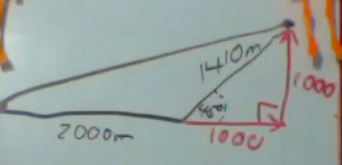Then we use the Pythagorean theorem to find the total displacement.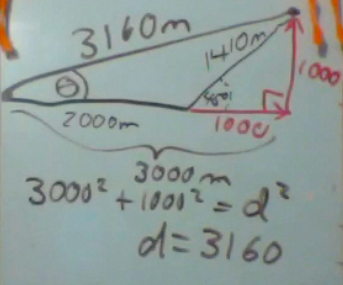However, displacement has a magnitude and a direction.  How do we find the angle θ?  We use the ArcTAN function.

The side opposite θ is 1000, and the adjacent side is 3000.  So we take the ArcTAN of (1/3) to get 18°